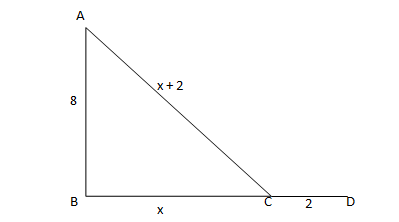• This is an assessment test.
• These tests focus on geometry and mensuration and are meant to indicate your preparation level for the subject.
• Kindly take the tests in this series with a pre-defined schedule.

## Geometry and Mensuration: Test 18

Congratulations - you have completed Geometry and Mensuration: Test 18. You scored %%SCORE%% out of %%TOTAL%%. You correct answer percentage: %%PERCENTAGE%% . Your performance has been rated as %%RATING%%
 Question 1
An angle is equal to 1/3rd of its supplement. Find its measure.
 A 60o B 80o C 90o D 45o
Question 1 Explanation:
$\displaystyle \begin{array}{l}\begin{array}{*{35}{l}} Let\text{ }that\text{ }angle\text{ }be\text{ }p \\ Supplement\text{ }of\text{ }this\text{ }angle\text{ }=\text{ }180-p \\ \end{array}\\Therefore\,\,\,p=\frac{1}{3}\,\left( 180-p \right)\\\Rightarrow 3p=180-p\\\Rightarrow p={{45}^{o}}\end{array}$
 Question 2
In a triangle ABC, ∠A = 90o and D is mid-point of AC. The value of BC2 – BD2 is equal to
Question 2 Explanation:
BC2 = AB2 + AC2
BC2 – BD2 = AB2 + AC2 – AB2 –AD2
 Question 3
The in-radius of an equilateral triangle is of length 3 cm. Then the length of each of its medians is
 A 12 cm B 9/2 cm C 4 cm D 9 cm
Question 3 Explanation:In equilateral triangle centroid, incentre,
orthocenter coincide at the same points
Therefore Height= Median= 3×3= 9 cm.
 Question 4
If ABC is an equilateral triangle and D is a point on BC such that AD⊥BC ,then
 A AB: BD= 1: 1 B AB: BD= 1: 2 C AB: BD= 2: 1 D AB: BD= 3: 2
Question 4 Explanation:Let AB = 2 unit.
As the triangle is an equilateral triangle, the perpendicular from A will bisect BC. (in this case at D) BD = 1 unit.
Required ratio AB: BD= 2: 1.
Correct option is (c)
 Question 5
A ladder leans against a vertical wall. The top of the ladder is 8m above the ground. When the bottom of the ladder is moved 2 m farther away from the wall, the top of the ladder rests against the foot of the wall. What is the length of the ladder?
 A 10 m B 15 m C 20 m D 17 m
Question 5 Explanation:Let AB be the wall and AC is the ladder with C as the foot of the ladder.
Let BC = x and CD = 2. So the length of the ladder is x + 2 i.e. AC = x + 2.
Now in ΔABC, we have (x + 2)2 = x2 + 64
=> x2 + 4 + 4x = x2 + 64
=> 4x = 60 => x = 15m.
Hence the length of the ladder = 15 + 2 = 17m.
Once you are finished, click the button below. Any items you have not completed will be marked incorrect.
There are 5 questions to complete.
 ← List →